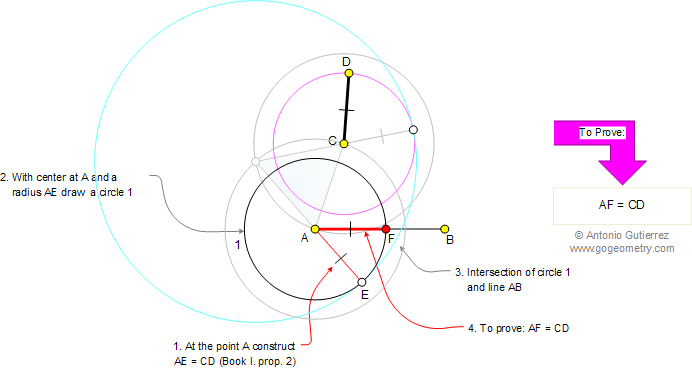Euclid's Elements Book I, Proposition 3: Given two unequal straight lines, to cut off from the greater a straight line equal to the less

Let AB and CD be the two given unequal straight lines, and let AB be the greater of them. Thus it is required to cut off from AB a straight line equal to CD.ConstructionThe Elements: Books I-XIII

 Euclid's Elements is the oldest mathematical and geometric treatise consisting of 13 books written by Euclid in Alexandria c. 300 BC. It is a collection of definitions, postulates, axioms, 467 propositions (theorems and constructions), and mathematical proofs of the propositions.